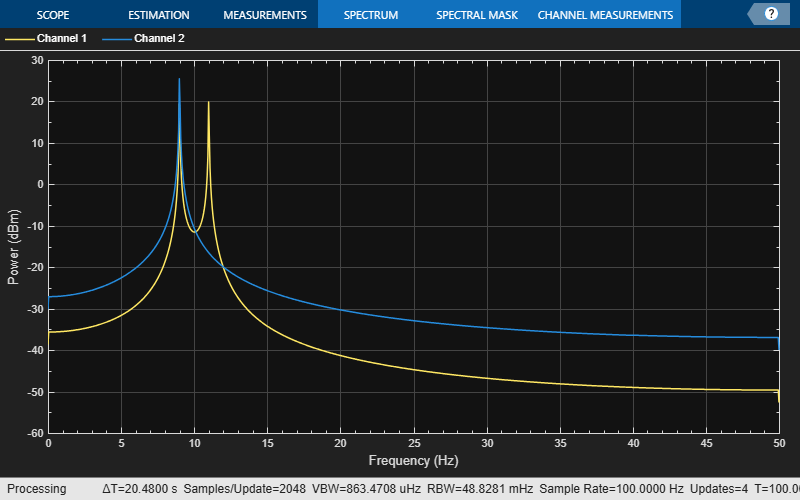ssbmod

Single sideband amplitude modulation

Syntax

y = ssbmod(x,Fc,Fs)
y = ssbmod(x,Fc,Fs,ini_phase)
y = ssbmod(x,fc,fs,ini_phase,'upper')

Description

y = ssbmod(x,Fc,Fs) uses the message signal x to modulate a carrier signal with frequency Fc (Hz) using single sideband amplitude modulation in which the lower sideband is the desired sideband. The generated output y is a single side band signal with a suppressed carrier. The carrier signal and x have sample frequency Fs (Hz). The modulated signal has zero initial phase.

y = ssbmod(x,Fc,Fs,ini_phase) specifies the initial phase of the modulated signal in radians.

y = ssbmod(x,fc,fs,ini_phase,'upper') uses the upper sideband as the desired sideband.

Examples

collapse all

Set the sample rate to 100 Hz. Create a time vector 100 seconds long.

fs = 100;
t = (0:1/fs:100)';

Set the carrier frequency to 10 Hz. Generate a sinusoidal signal.

fc = 10;
x = sin(2*pi*t);

Modulate x using single- and double-sideband AM.

ydouble = ammod(x,fc,fs);
ysingle = ssbmod(x,fc,fs);

Create a spectrum analyzer object to plot the spectra of the two signals. Plot the spectrum of the double-sideband signal.

sa = dsp.SpectrumAnalyzer('SampleRate',fs, ...
'PlotAsTwoSidedSpectrum',false, ...
'YLimits',[-60 40]);
step(sa,ydouble)Plot the single-sideband spectrum.

step(sa,ysingle)